# Chapter 7 Exploratory Data Analysis

Author: CW
Status: On-going
Reviewer:

## 7.1 Introduction

### 7.1.1 Prerequisites

``````library(tidyverse)
library(nycflights13)``````

## 7.3 Variation

``````ggplot(data = diamonds) +
geom_bar(mapping = aes(x = cut))``````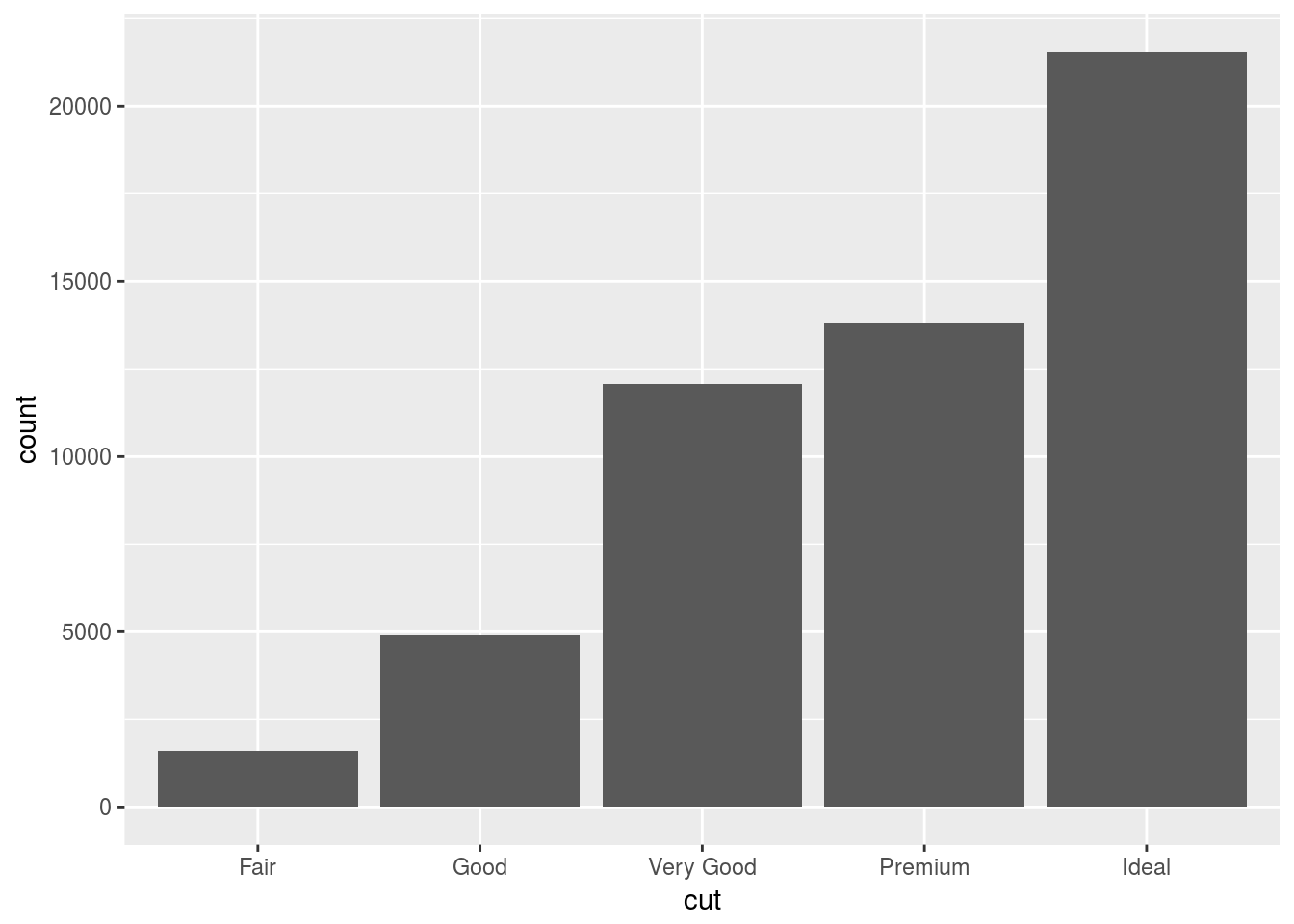``````smaller <- diamonds %>%
filter(carat < 3)``````

### 7.3.4 Exercises

1. Explore the distribution of each of the `x`, `y`, and `z` variables in diamonds. What do you learn? Think about a diamond and how you might decide which dimension is the length, width, and depth.
``````# remove false data points
diamonds <- diamonds %>% filter(2 < y & y < 20 & 2 < x & 2 < z & z < 20)
ggplot(diamonds) +
geom_freqpoly(aes(x = x), binwidth = 0.01)``````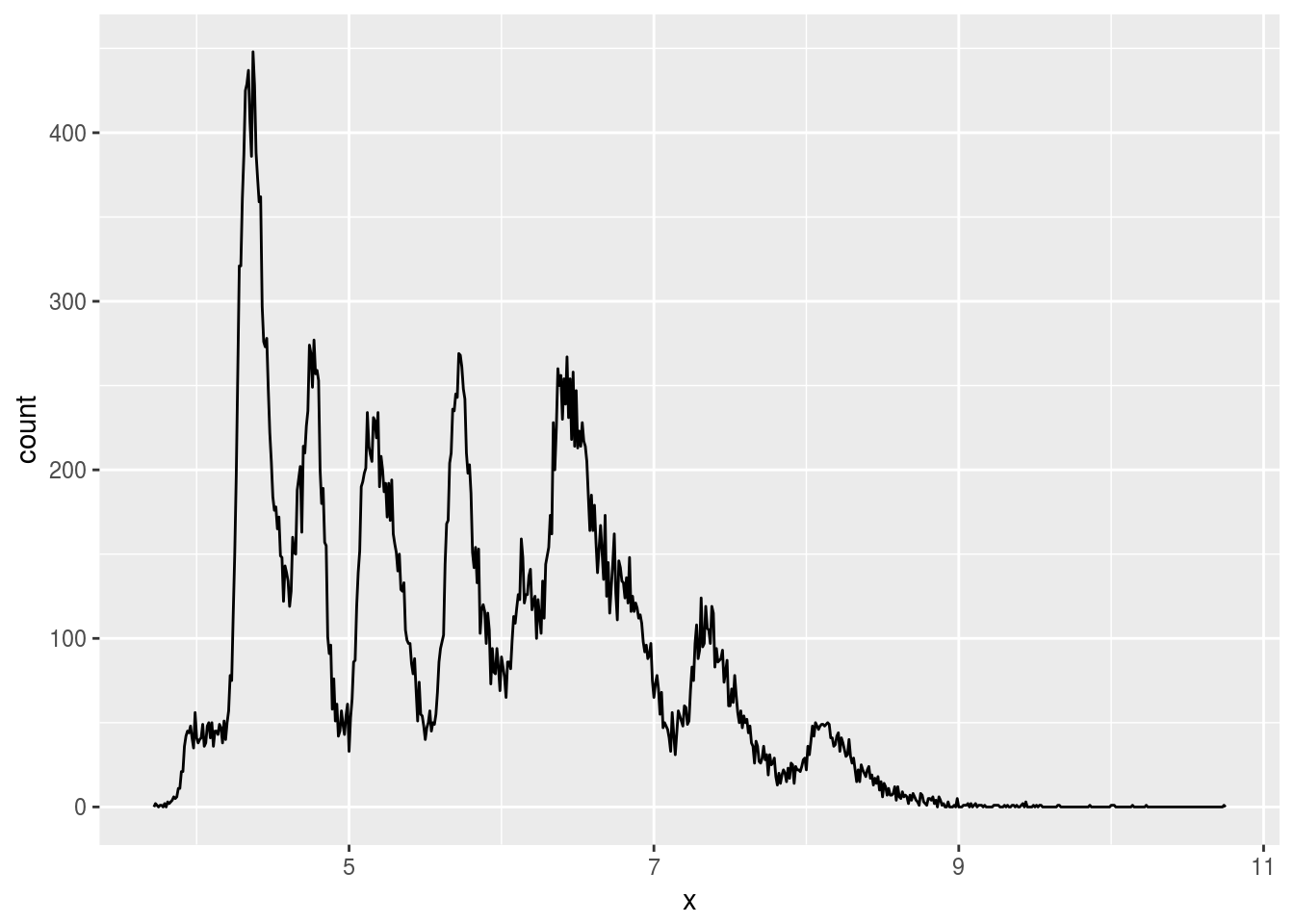``````ggplot(diamonds) +
geom_freqpoly(aes(x = y), binwidth = 0.01)``````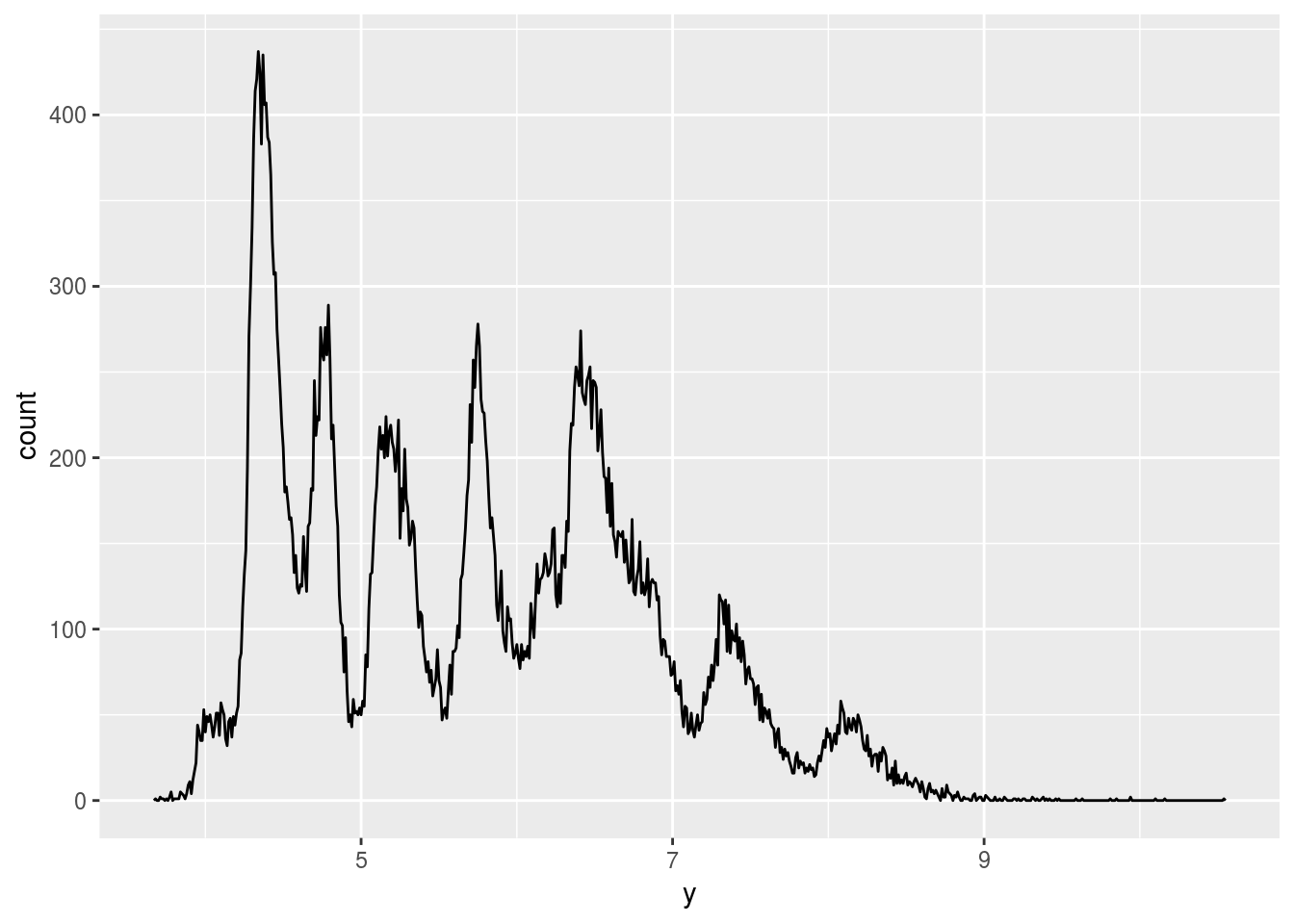``````ggplot(diamonds) +
geom_freqpoly(aes(x = z), binwidth = 0.01)``````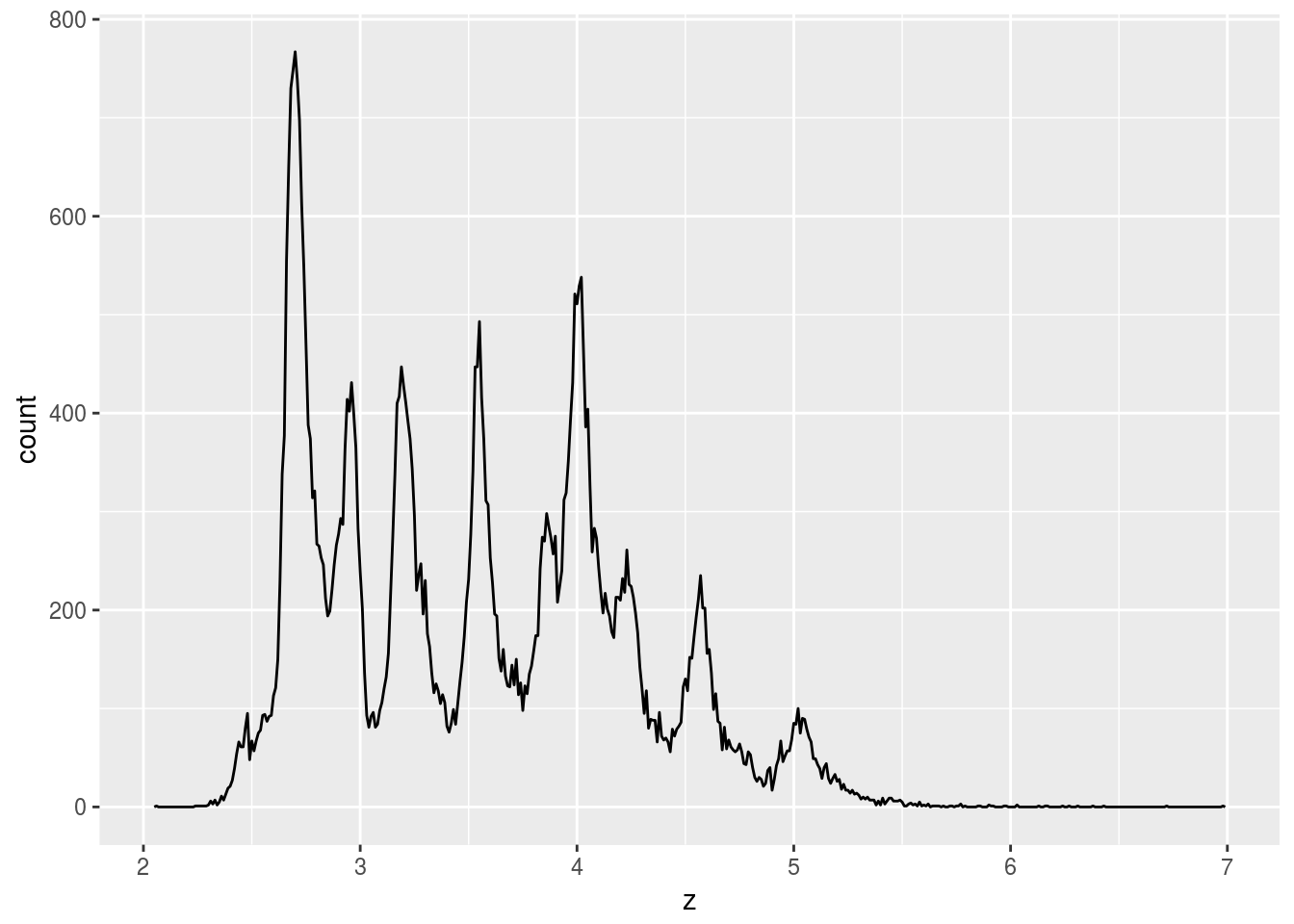``````# x and y often share value
ggplot(diamonds) +
geom_point(aes(x = x, y = y)) +
geom_point(aes(x = x, y = z), color = "blue") +
coord_fixed()``````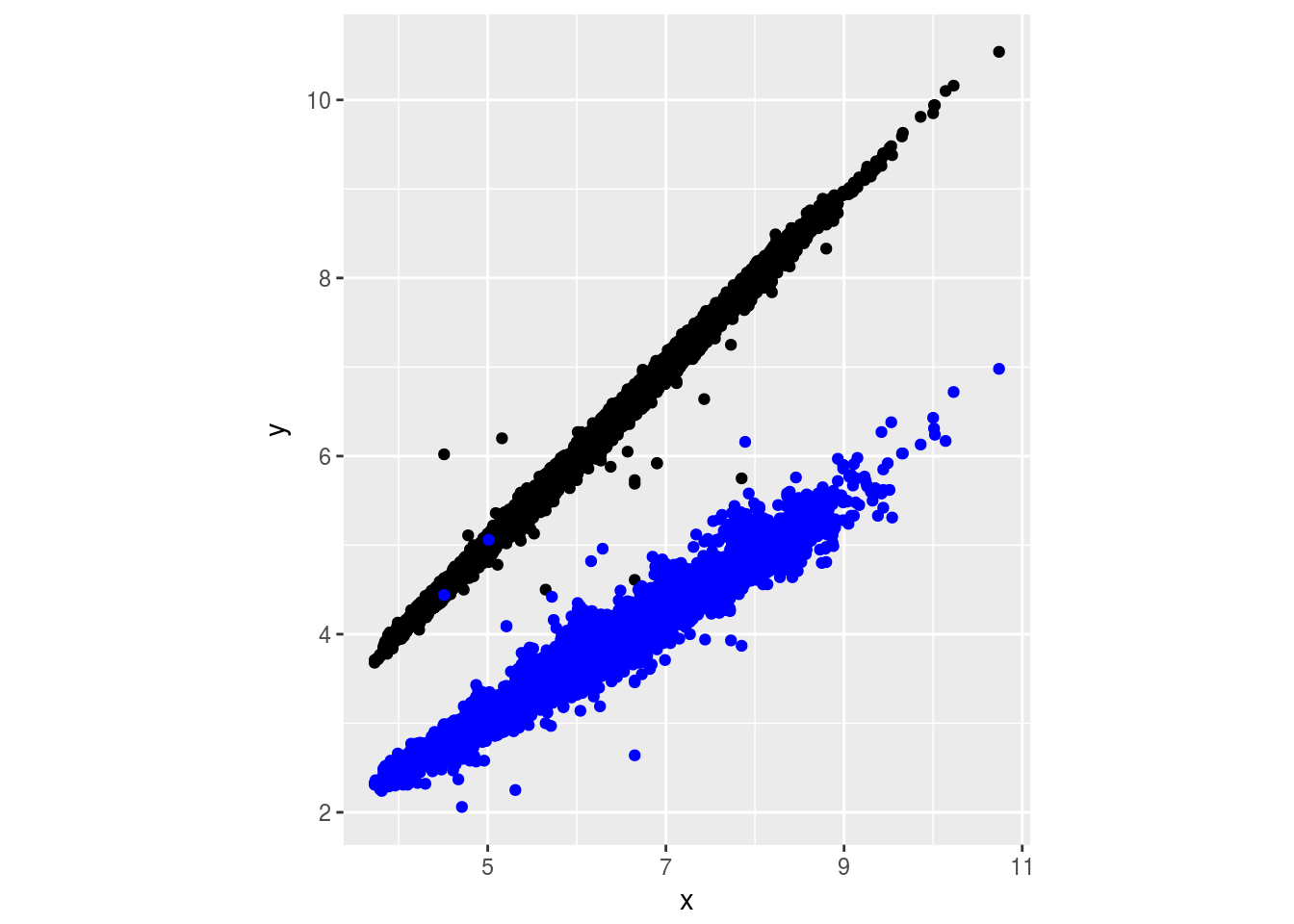Seems like `x` and `y` should be length and width, and `z` is depth.

1. Explore the distribution of `price`. Do you discover anything unusual or surprising? (Hint: Carefully think about the `binwidth` and make sure you try a wide range of values.)
``````# remove false data points
diamonds <- diamonds %>% filter(2 < y & y < 20 & 2 < x & 2 < z & z < 20)
ggplot(diamonds) +
geom_freqpoly(aes(x = price), binwidth = 10) +
xlim(c(1000, 2000))``````
``## Warning: Removed 44207 rows containing non-finite values (stat_bin).``
``## Warning: Removed 2 rows containing missing values (geom_path).``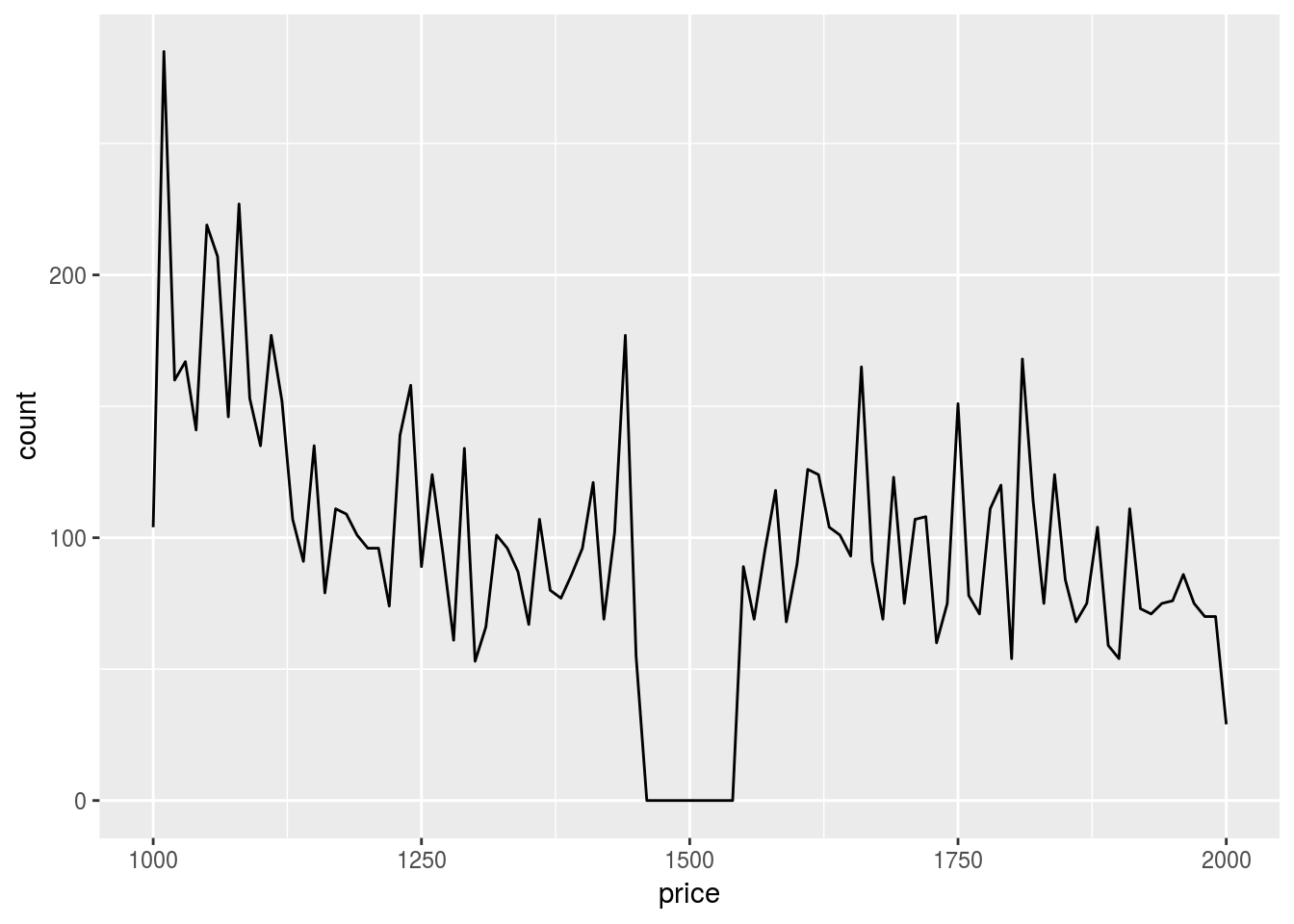Somehow we don’t have diamonds that are priced around \$1500.

1. How many diamonds are 0.99 carat? How many are 1 carat? What do you think is the cause of the difference?
``diamonds %>% filter(carat == 0.99) %>% count()``
``````## # A tibble: 1 x 1
##       n
##   <int>
## 1    23``````
``diamonds %>% filter(carat == 1) %>% count()``
``````## # A tibble: 1 x 1
##       n
##   <int>
## 1  1556``````
``````ggplot(diamonds) +
geom_histogram(aes(x = carat), binwidth = 0.01) +
xlim(c(0.97, 1.03))``````
``## Warning: Removed 48599 rows containing non-finite values (stat_bin).``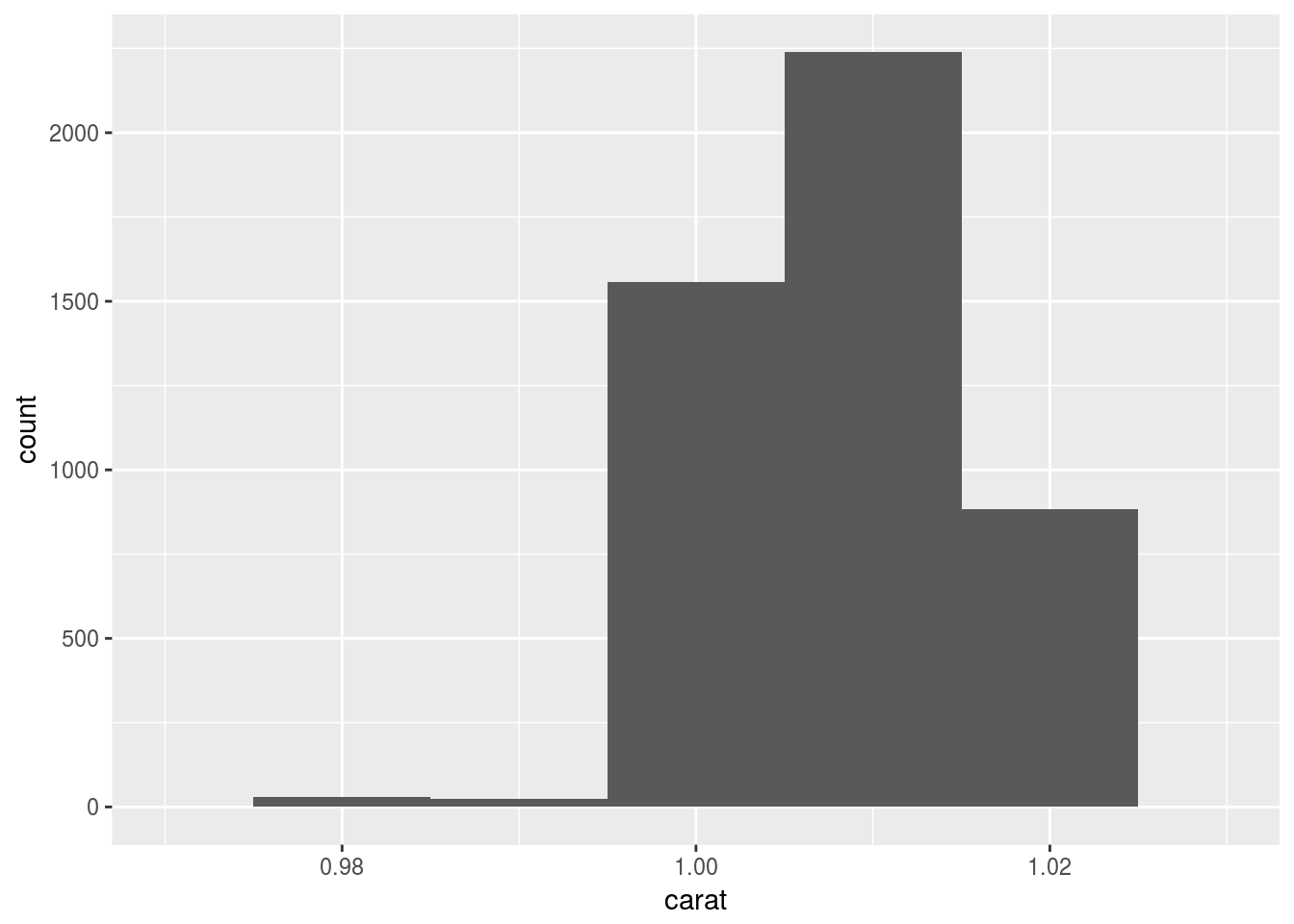There are much more diamonds with 1 carat. I think it is because psychologically, 1 carat represent a whole new level from 0.99 carat, so for makers, it is little more material for much more value.

1. Compare and contrast `coord_cartesian()` vs `xlim()` or `ylim()` when zooming in on a histogram. What happens if you leave `binwidth` unset? What happens if you try and zoom so only half a bar shows?
``````ggplot(diamonds) +
geom_histogram(aes(x = carat)) +
xlim(c(0.97, 1.035))``````
``## `stat_bin()` using `bins = 30`. Pick better value with `binwidth`.``
``## Warning: Removed 48599 rows containing non-finite values (stat_bin).``
``## Warning: Removed 1 rows containing missing values (geom_bar).``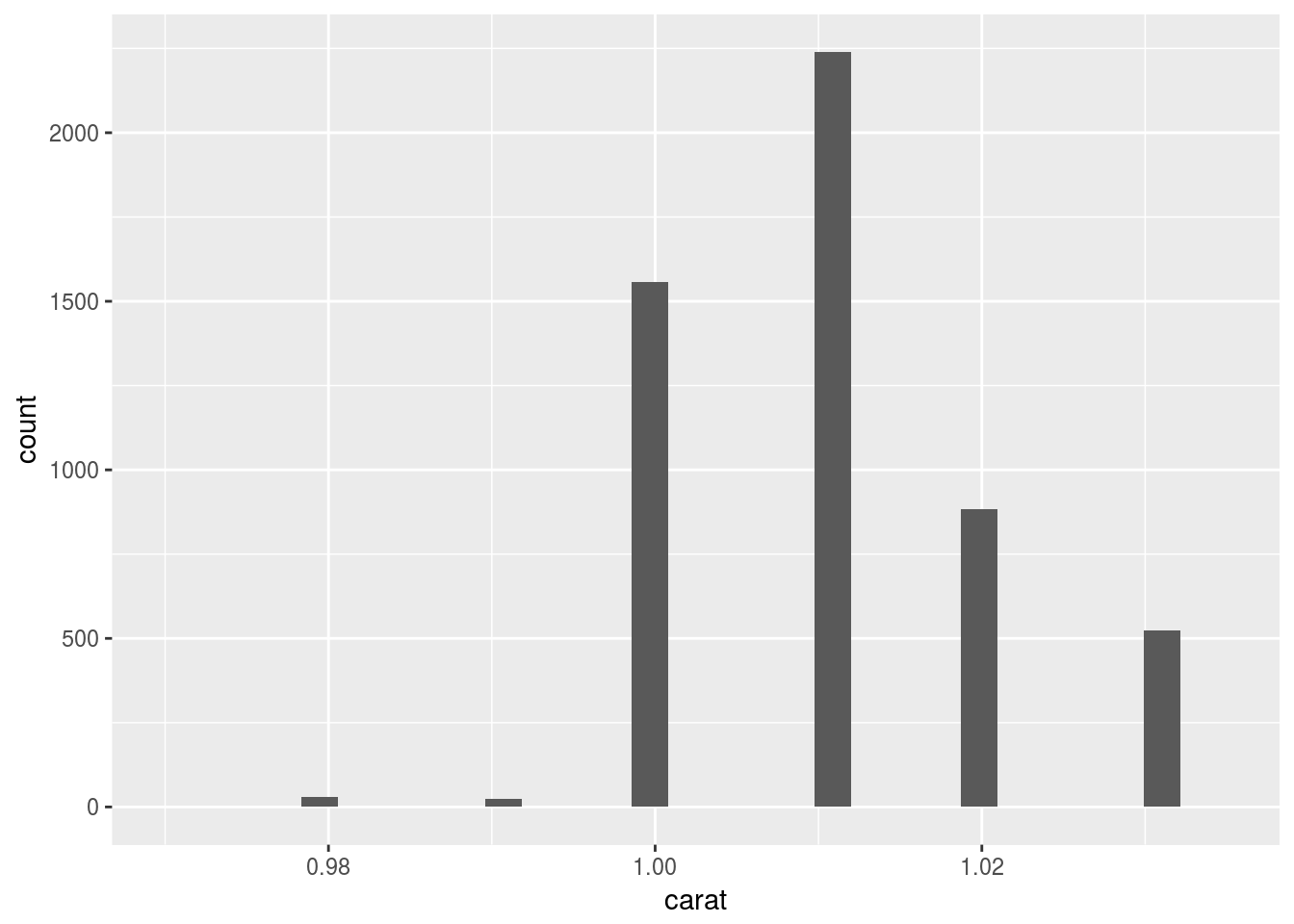``````ggplot(diamonds) +
geom_histogram(aes(x = carat)) +
coord_cartesian(xlim = c(0.97, 1.035))``````
``## `stat_bin()` using `bins = 30`. Pick better value with `binwidth`.``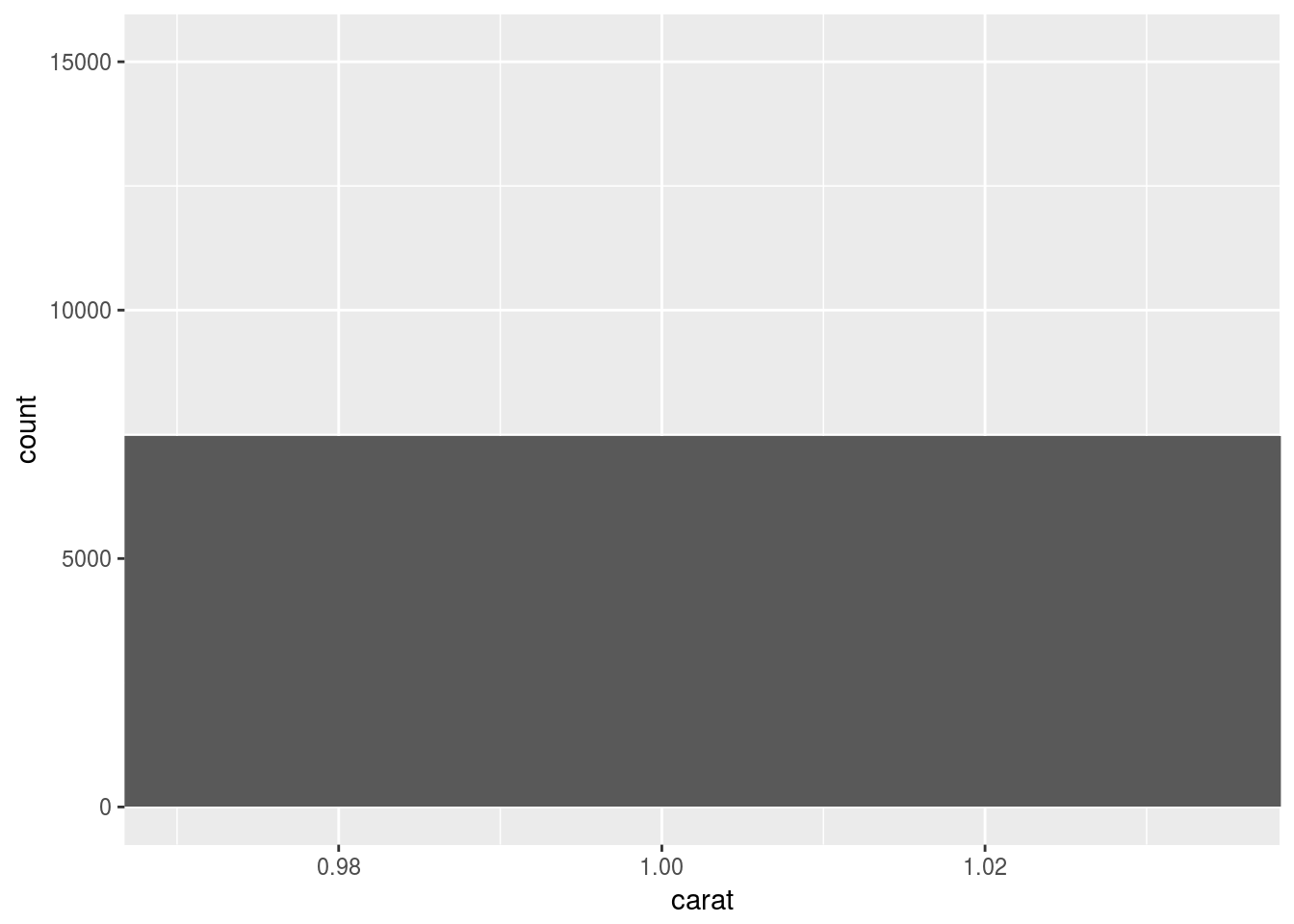``````ggplot(diamonds) +
geom_histogram(aes(x = carat), binwidth = 0.01) +
xlim(c(0.97, 1.035))``````
``## Warning: Removed 48599 rows containing non-finite values (stat_bin).``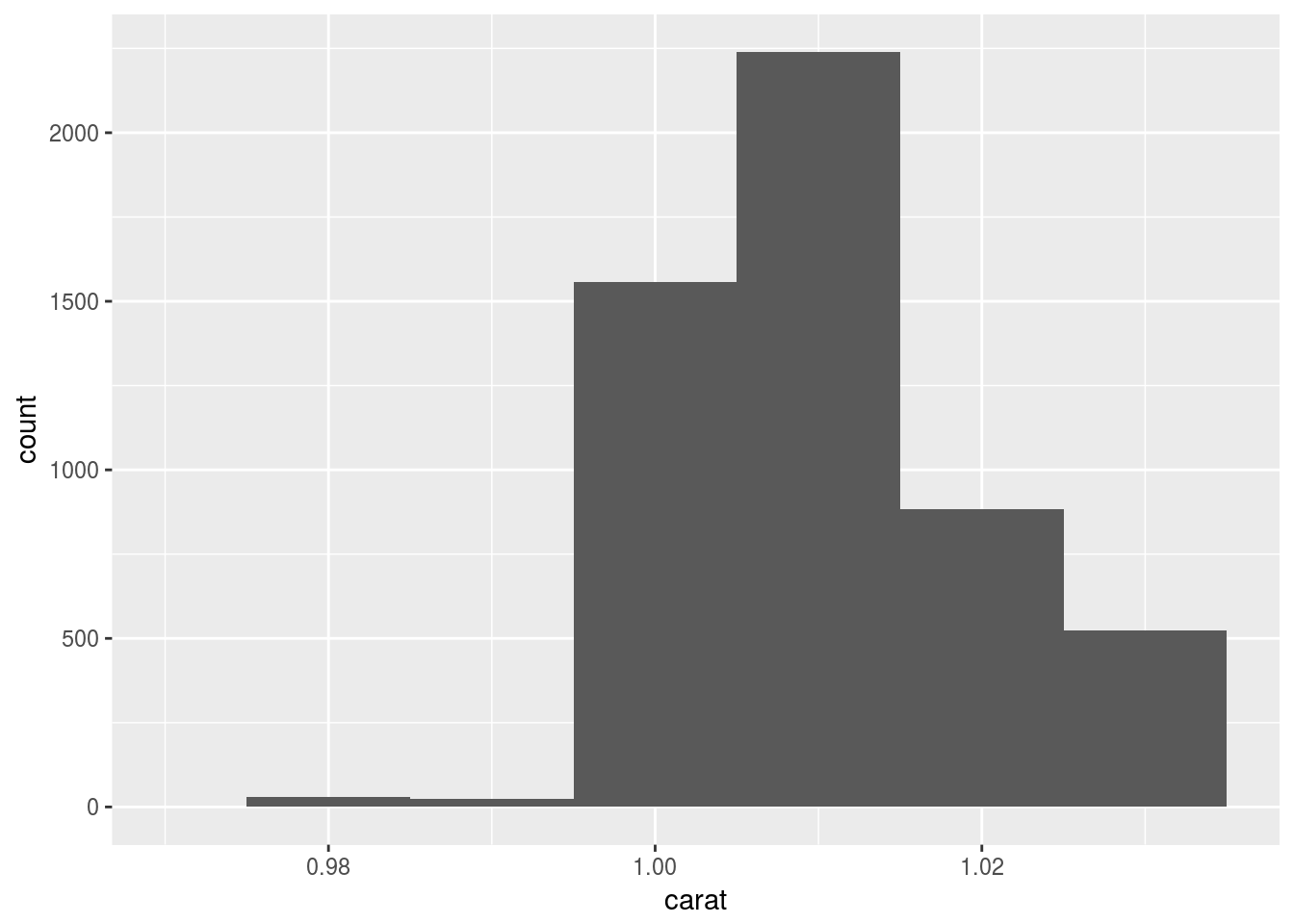``````ggplot(diamonds) +
geom_histogram(aes(x = carat), binwidth = 0.01) +
coord_cartesian(xlim = c(0.97, 1.035))``````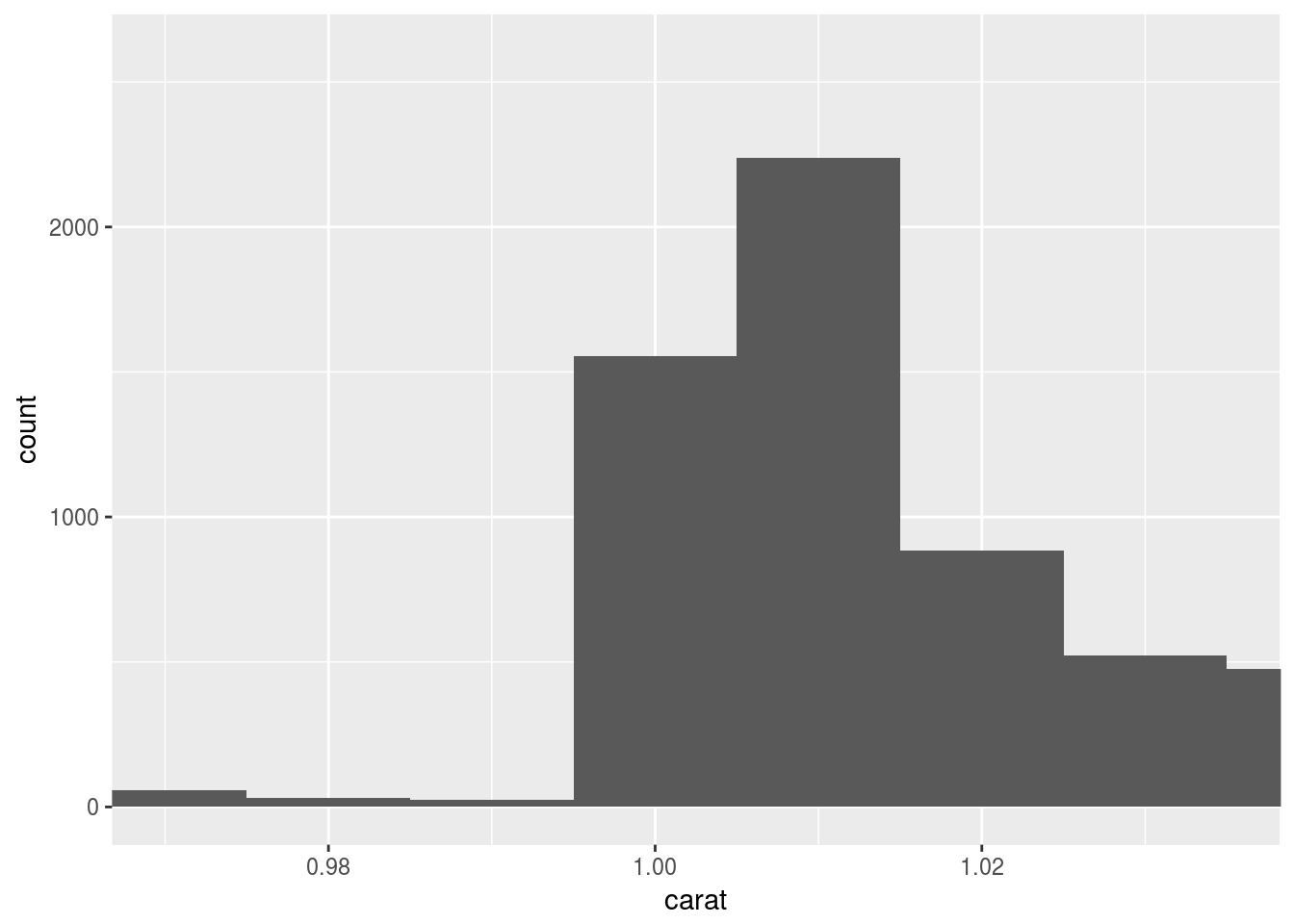`coord_cartesian()` plots and cuts, while `xlim()` cuts and plots. So `xlim()` does not show the half bar.

## 7.4 Missing values

### 7.4.1 Exercises

1. What happens to missing values in a histogram? What happens to missing values in a bar chart? Why is there a difference?
In a bar chart, `NA` is considered as just another category. In a histogram, `NA` is ignored because the x exis has order.
``````set.seed(0)
df <- tibble(norm = rnorm(100)) %>% mutate(inrange = ifelse(norm > 2, NA, norm))
ggplot(df) +
geom_histogram(aes(x = inrange))``````
``## `stat_bin()` using `bins = 30`. Pick better value with `binwidth`.``
``## Warning: Removed 2 rows containing non-finite values (stat_bin).``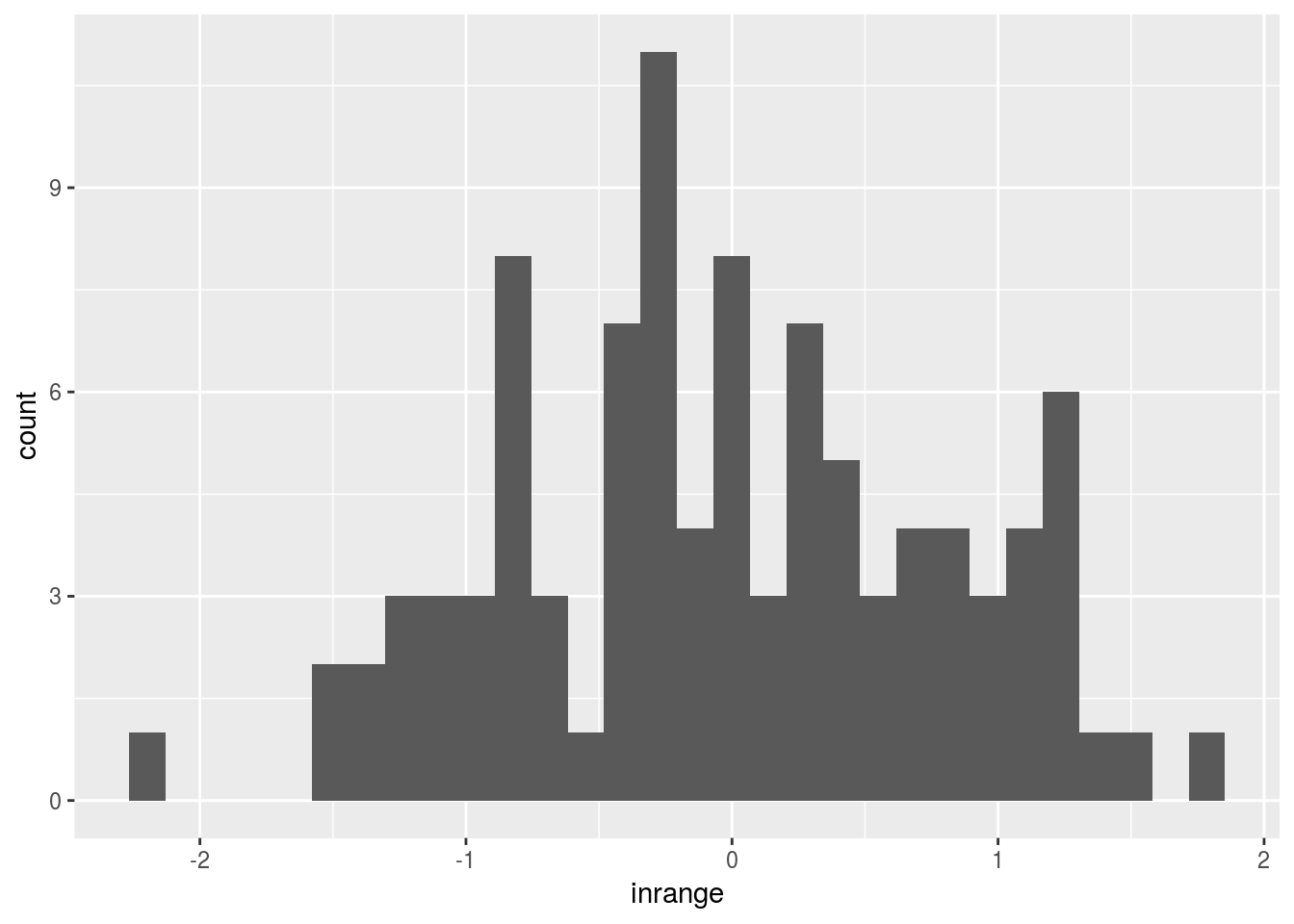`geom_histogram()` removed rows with `NA` values;

``````df <- diamonds %>% mutate(cut = as.factor(ifelse(y > 7, NA, cut)))
ggplot(df) + geom_bar(aes(x = cut))``````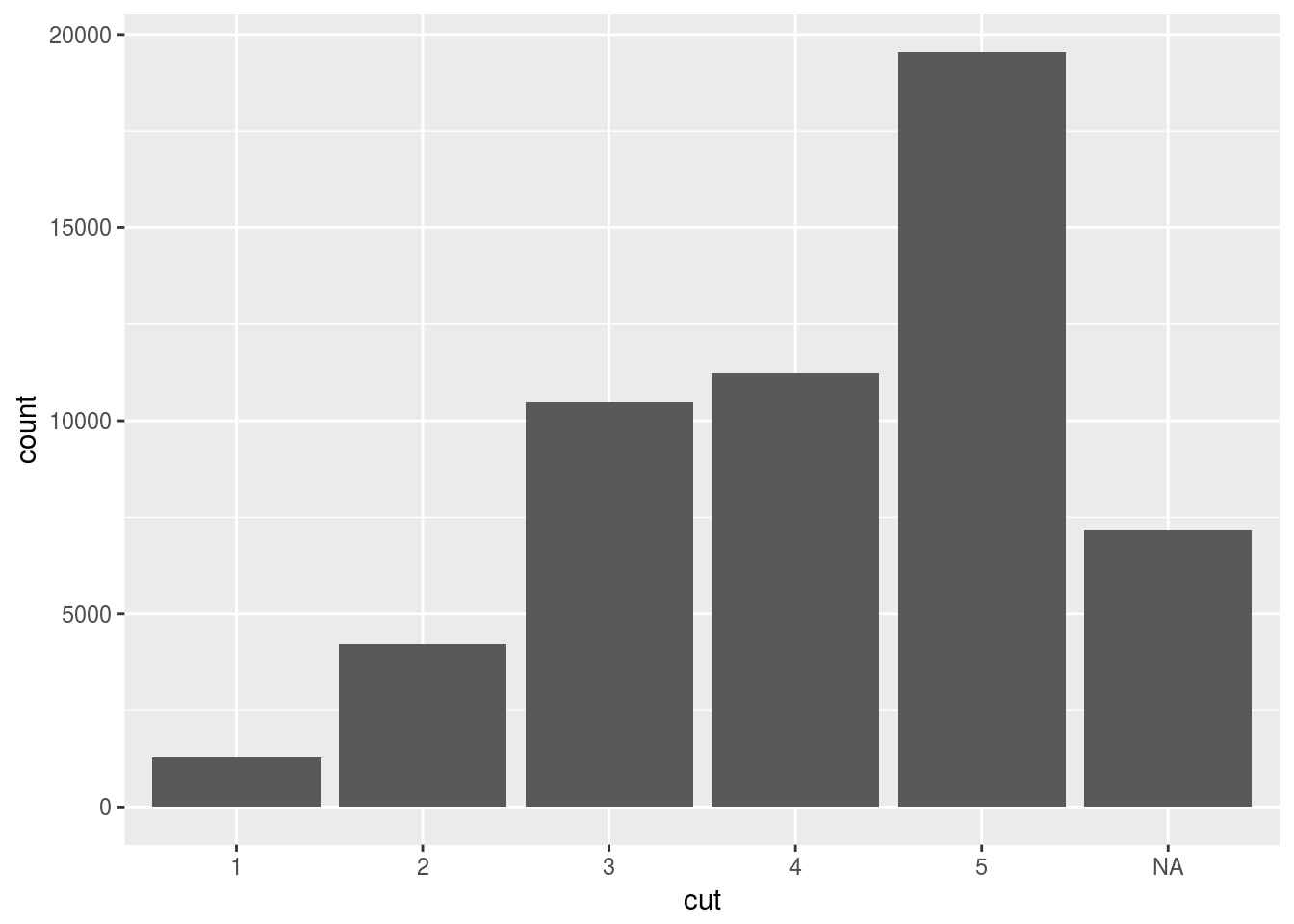Apparently `geom_bar()` doesn’t remove `NA`, but rather treat it as another factor or category.

1. What does `na.rm = TRUE` do in `mean()` and `sum()`?
To ignore `NA`s when calculating mean and sum.

## 7.5 Covariation

### 7.5.1 A categorical and continuous variable

#### 7.5.1.1 Exercises

1. Use what you’ve learned to improve the visualisation of the departure times of cancelled vs. non-cancelled flights.
``````flights %>%
mutate(cancelled = is.na(dep_time) | is.na(arr_time)) %>%
ggplot() +
geom_boxplot(aes(x = cancelled, y = dep_time))``````
``## Warning: Removed 8255 rows containing non-finite values (stat_boxplot).``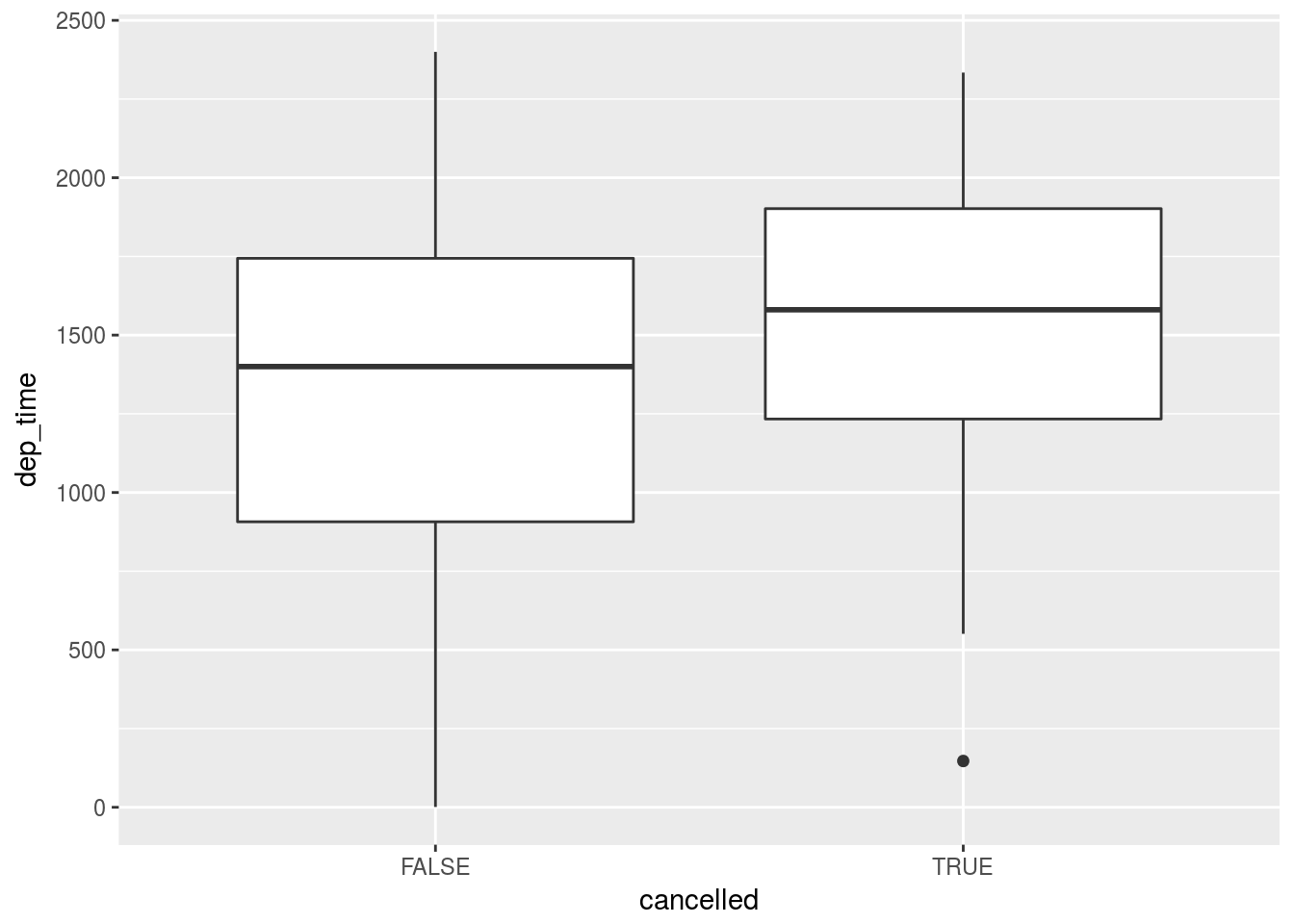``````flights %>%
mutate(cancelled = is.na(dep_time) | is.na(arr_time)) %>%
filter(cancelled) %>%
select(dep_time)``````
``````## # A tibble: 8,713 x 1
##    dep_time
##       <int>
##  1     2016
##  2       NA
##  3       NA
##  4       NA
##  5       NA
##  6     2041
##  7     2145
##  8       NA
##  9       NA
## 10       NA
## # ... with 8,703 more rows``````

Puzzled by this question: how do we have departure times of cancelled flights?

1. What variable in the diamonds dataset is most important for predicting the price of a diamond? How is that variable correlated with cut? Why does the combination of those two relationships lead to lower quality diamonds being more expensive?
``````ggplot(diamonds) +
geom_point(aes(x = carat, y = price), color = "blue", alpha = 0.5)``````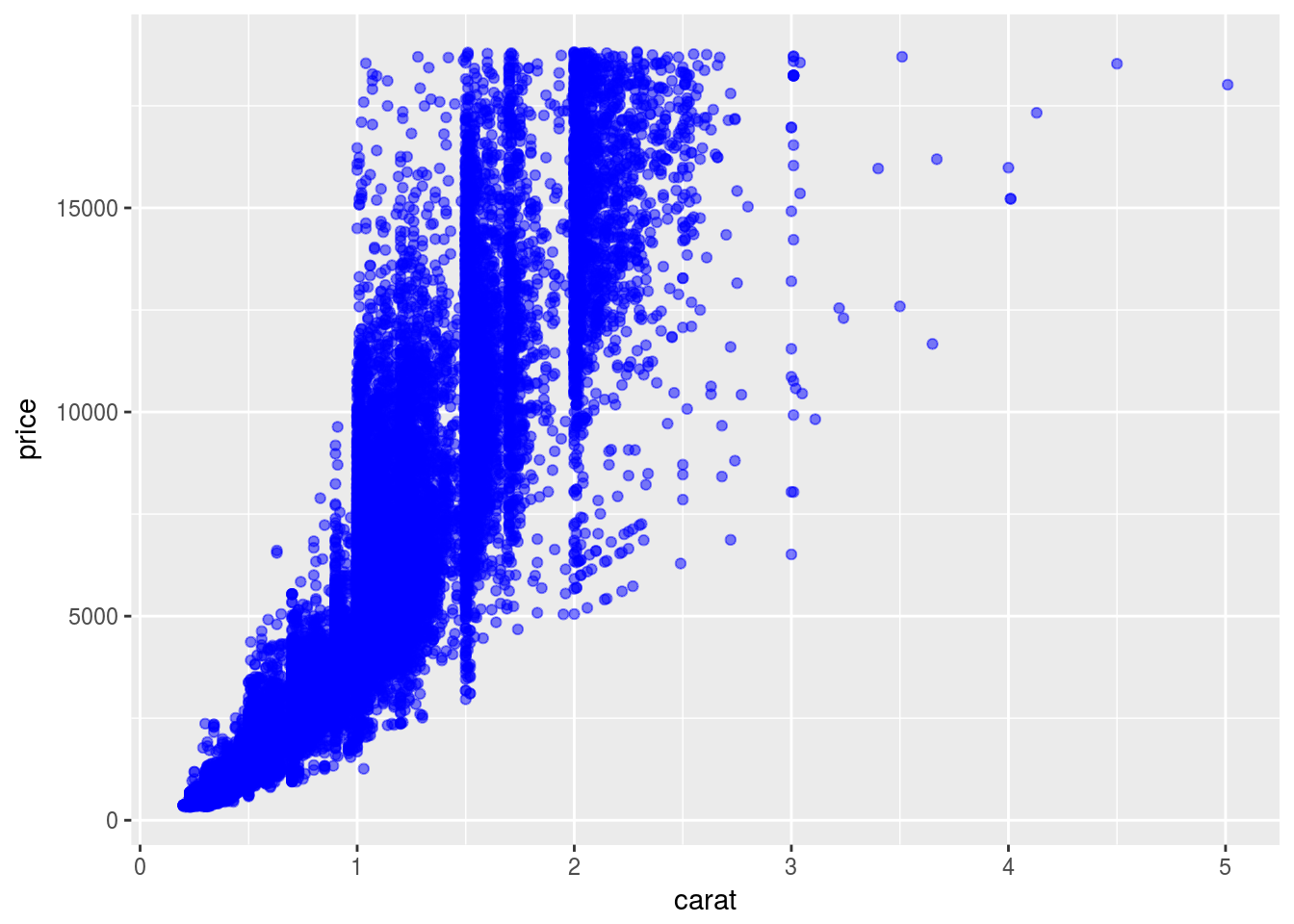``````ggplot(diamonds) +
geom_point(aes(x = depth, y = price), color = "red", alpha = 0.5)``````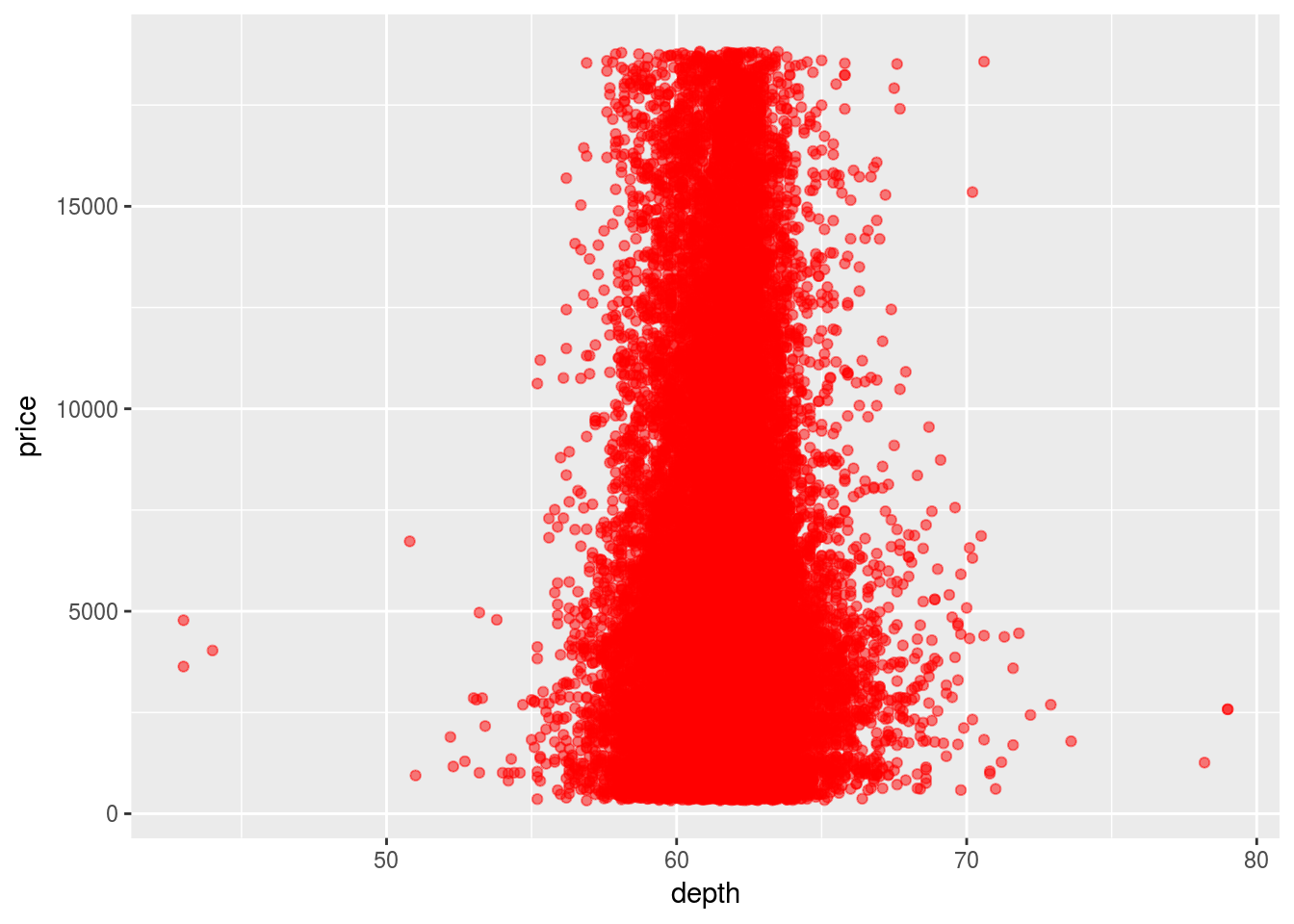``````ggplot(diamonds) +
geom_point(aes(x = table, y = price), color = "red", alpha = 0.5)``````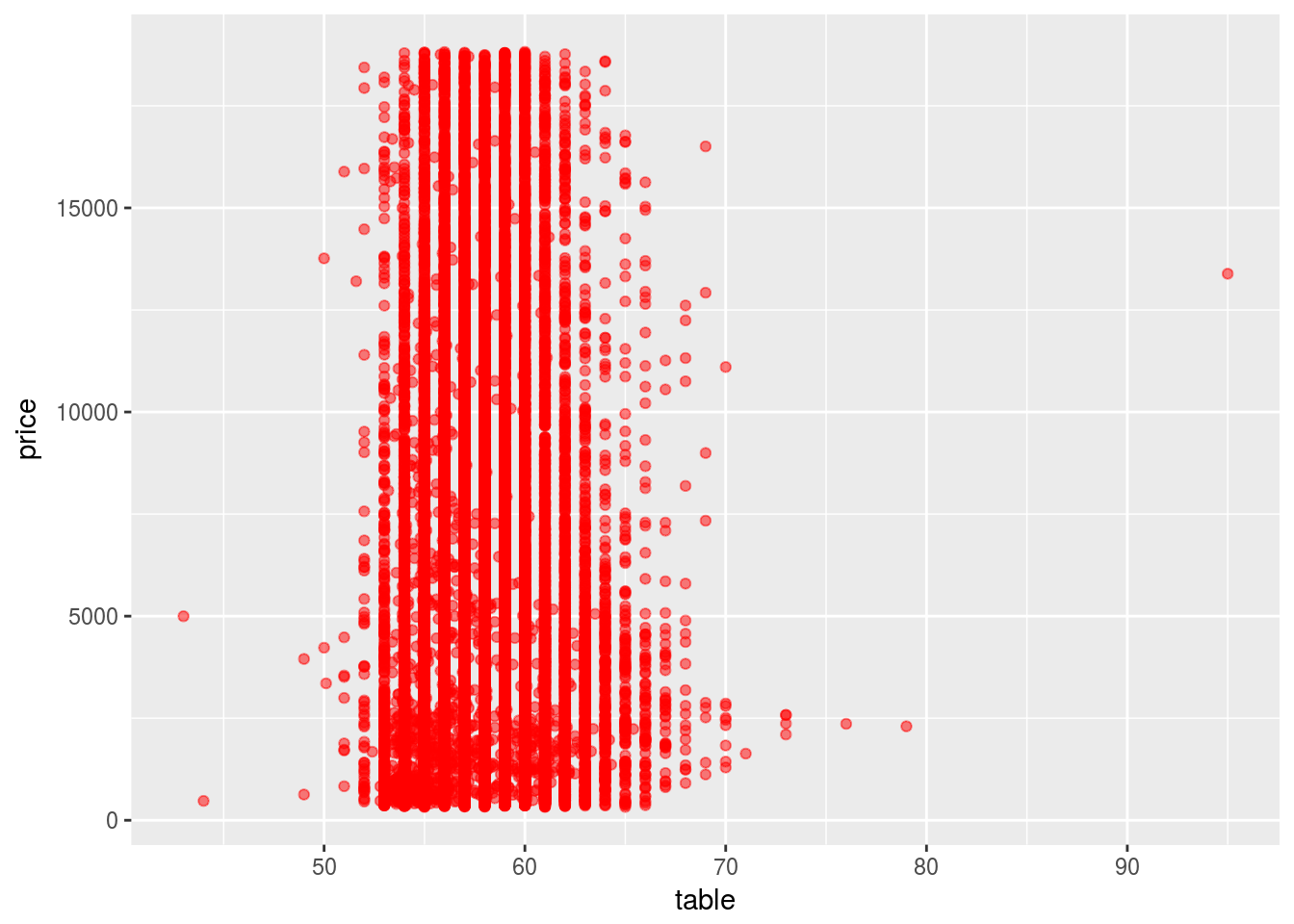``````ggplot(diamonds) +
geom_point(aes(x = x, y = price), color = "red", alpha = 0.5)``````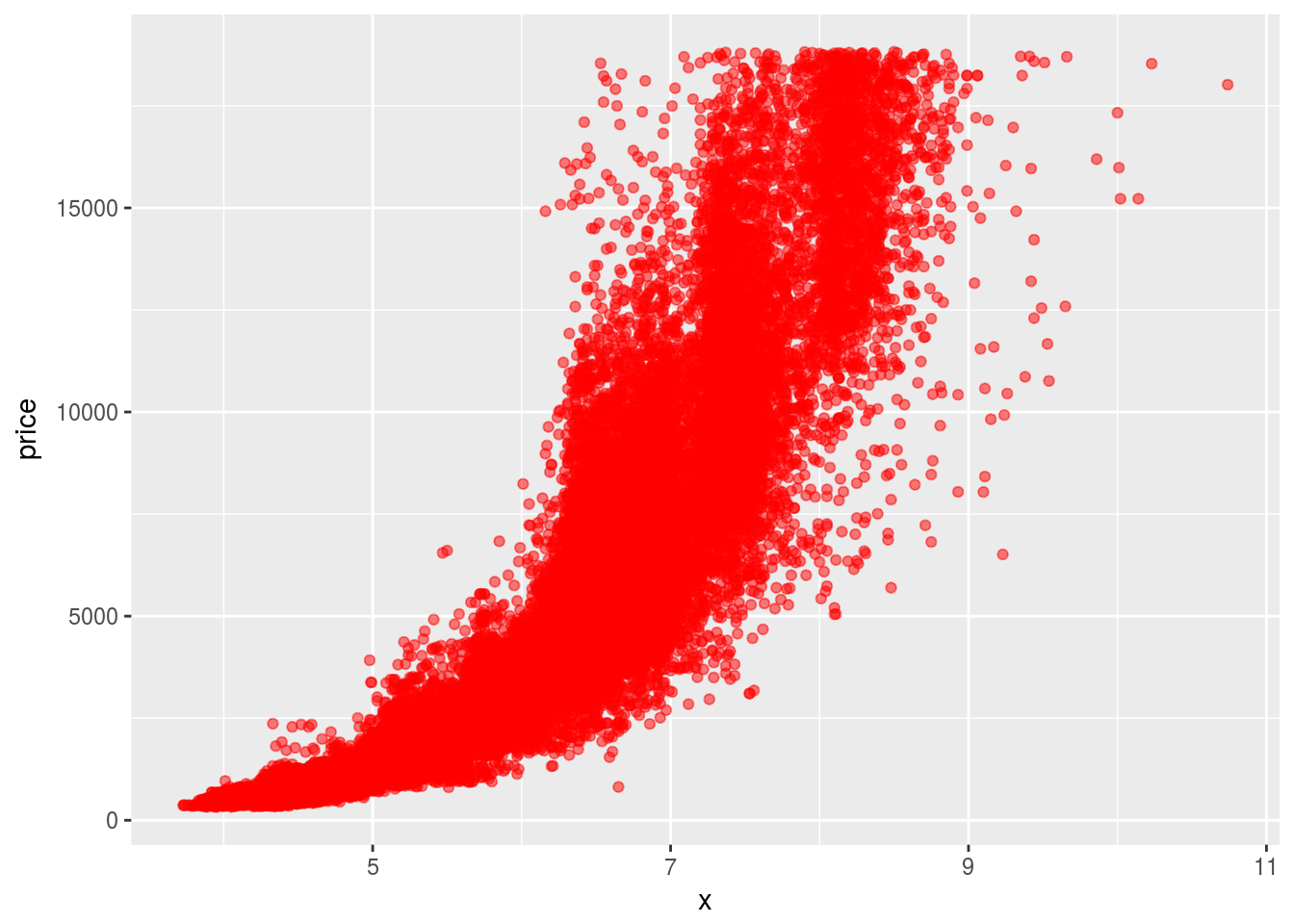``````ggplot(diamonds) +
geom_point(aes(x = z, y = price), color = "red", alpha = 0.5)``````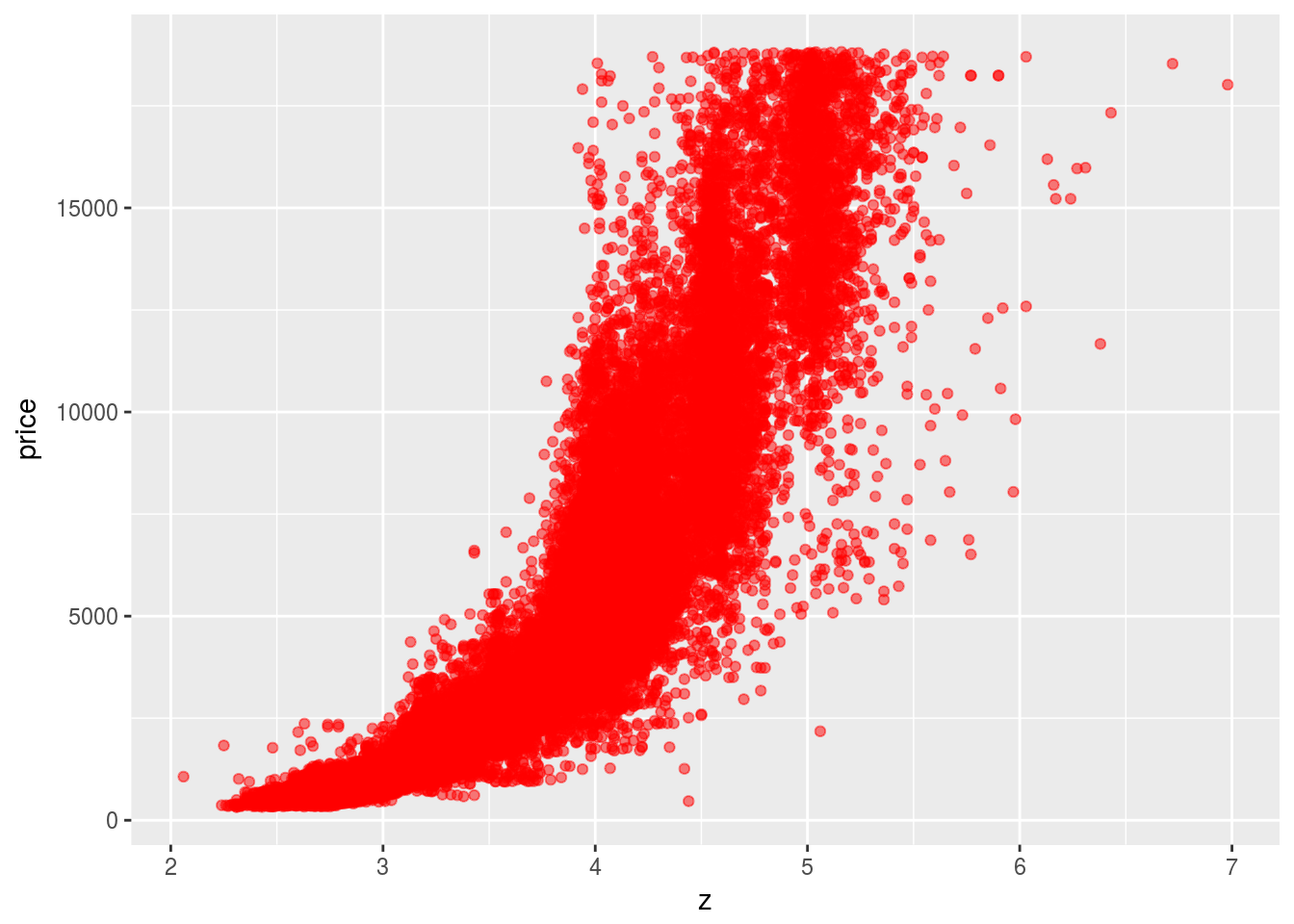Volumn and weight are two variables that is most important for predicting the price. Since volumn is highly correlated with weight, they can be considered to be one variable.

``````ggplot(diamonds) +
geom_boxplot(aes(x = cut, y = carat))``````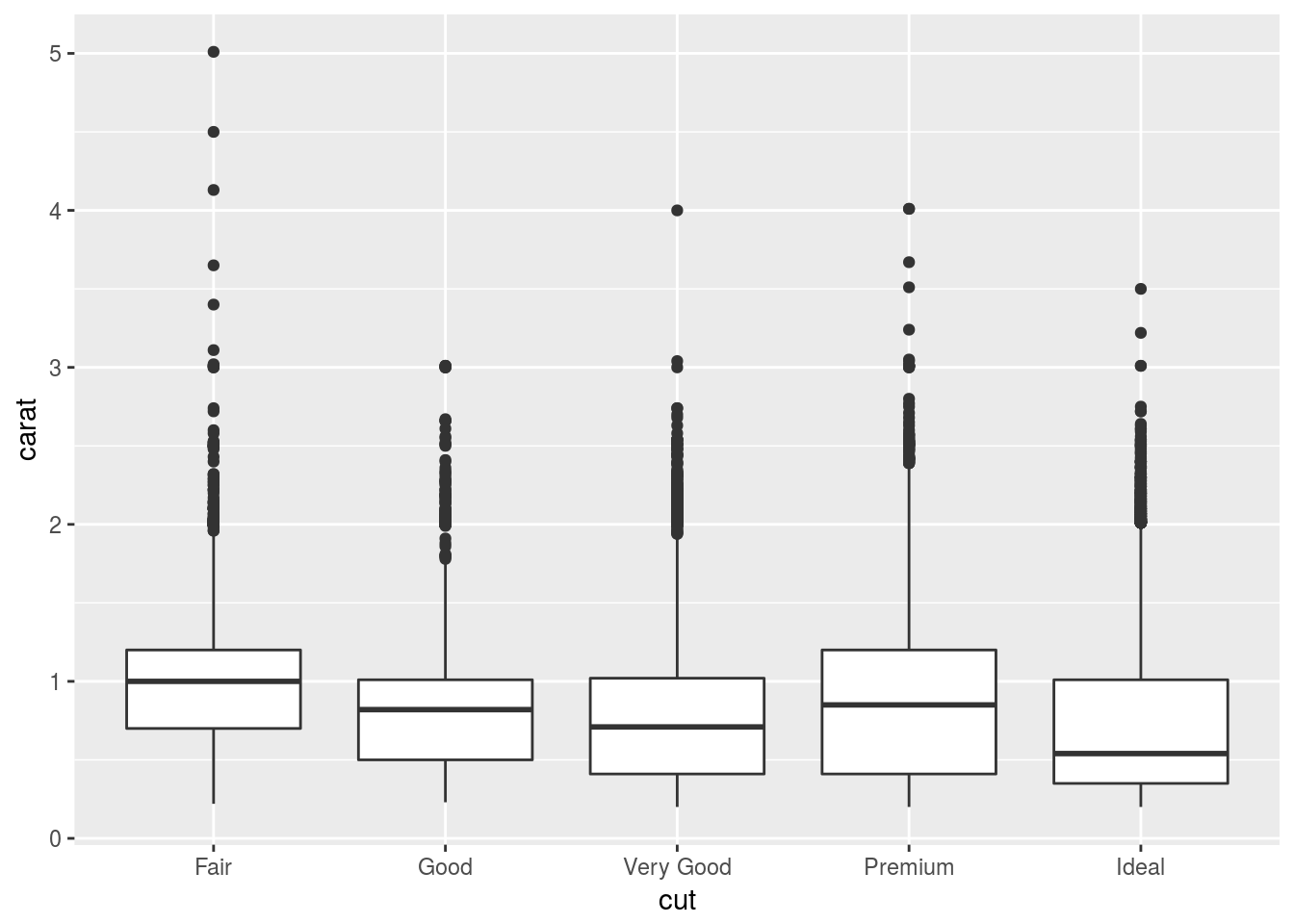Because better `cut` has lower `carat` which makes their `price` lower, so if we don’t look at `carat`, it would appear that better `cut` has lower `price`.

1. Install the `ggstance` package, and create a horizontal boxplot. How does this compare to using `coord_flip()`?
``library(ggstance)``
``````##
## Attaching package: 'ggstance'``````
``````## The following objects are masked from 'package:ggplot2':
##
##     geom_errorbarh, GeomErrorbarh``````
``ggplot(diamonds) + geom_boxplot(aes(x = cut, y = carat)) + coord_flip()``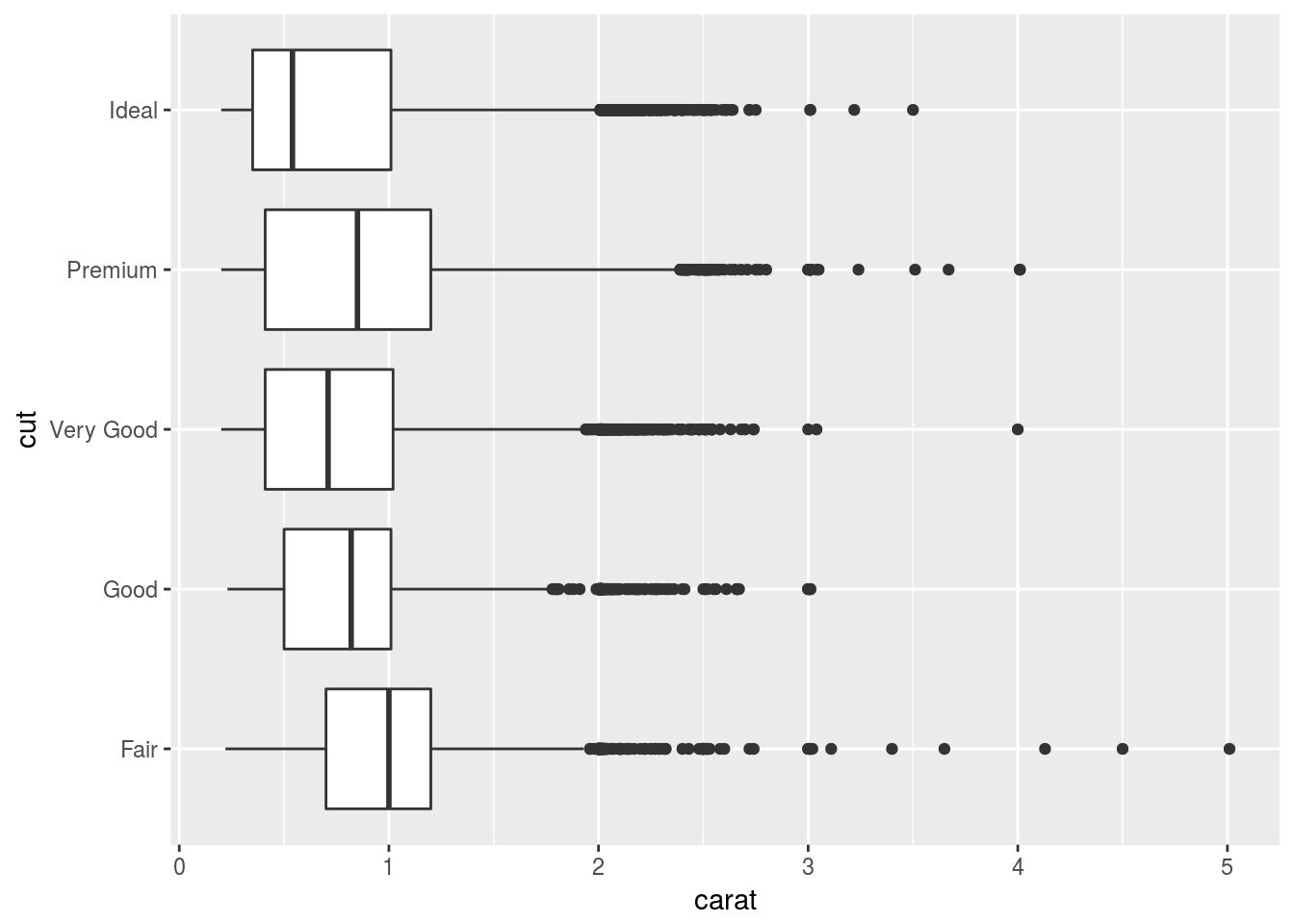``ggplot(diamonds) + geom_boxploth(aes(x = carat, y = cut))``Seems like the result is the same; but the call of the function seems more natural.

1. One problem with boxplots is that they were developed in an era of much smaller datasets and tend to display a prohibitively large number of “outlying values”. One approach to remedy this problem is the letter value plot. Install the `lvplot` package, and try using `geom_lv()` to display the distribution of `price` vs `cut`. What do you learn? How do you interpret the plots?
``````library(lvplot)
ggplot(diamonds) + geom_lv(aes(x = cut, y = price))``````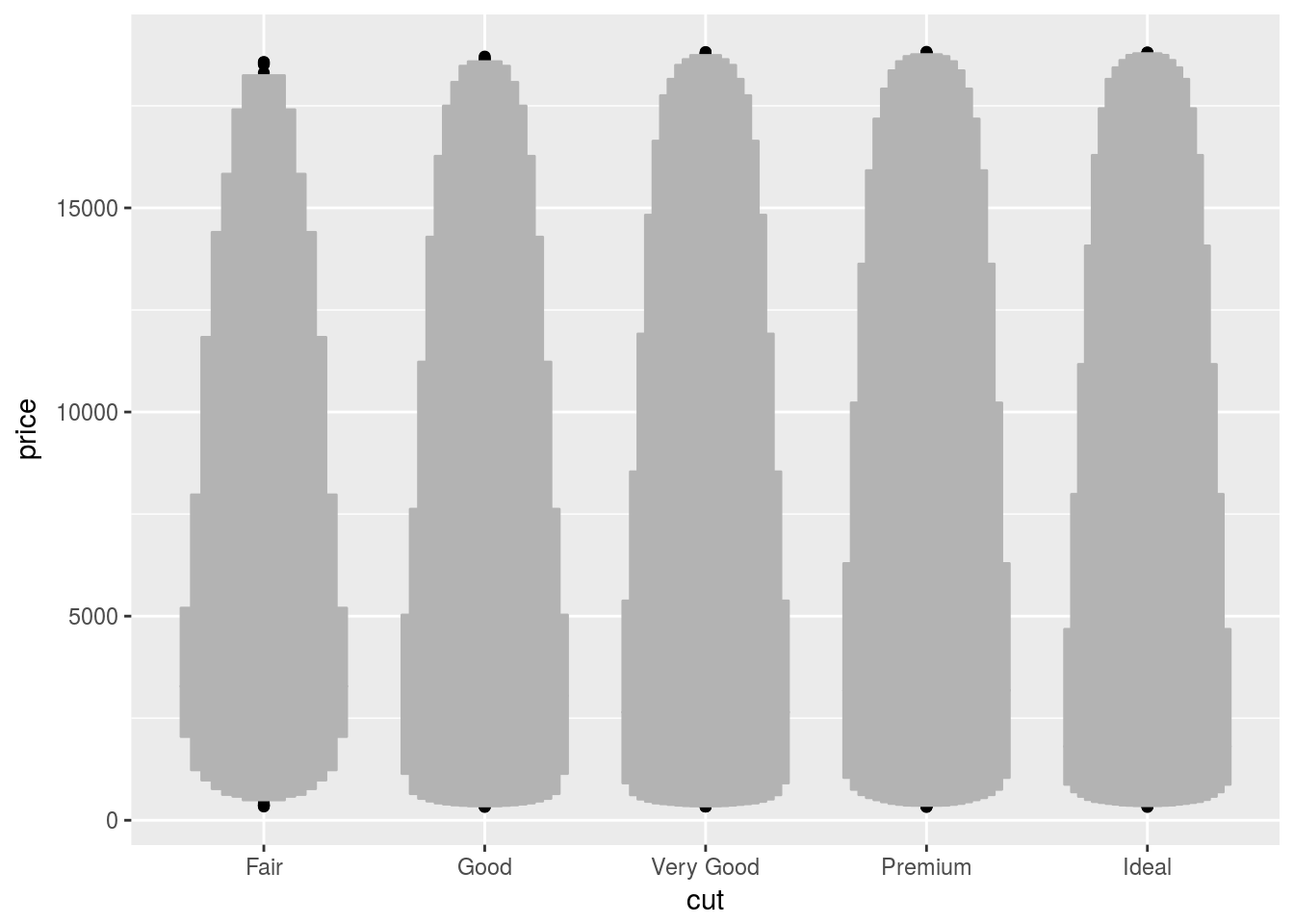While the boxplot only shows a few quantiles and outliers, the letter-value plot shows many quantiles.

1. Compare and contrast `geom_violin()` with a facetted `geom_histogram()`, or a coloured `geom_freqpoly()`. What are the pros and cons of each method?
``````ggplot(diamonds) +
geom_histogram(aes(x = price)) +
facet_wrap(~cut)``````
``## `stat_bin()` using `bins = 30`. Pick better value with `binwidth`.``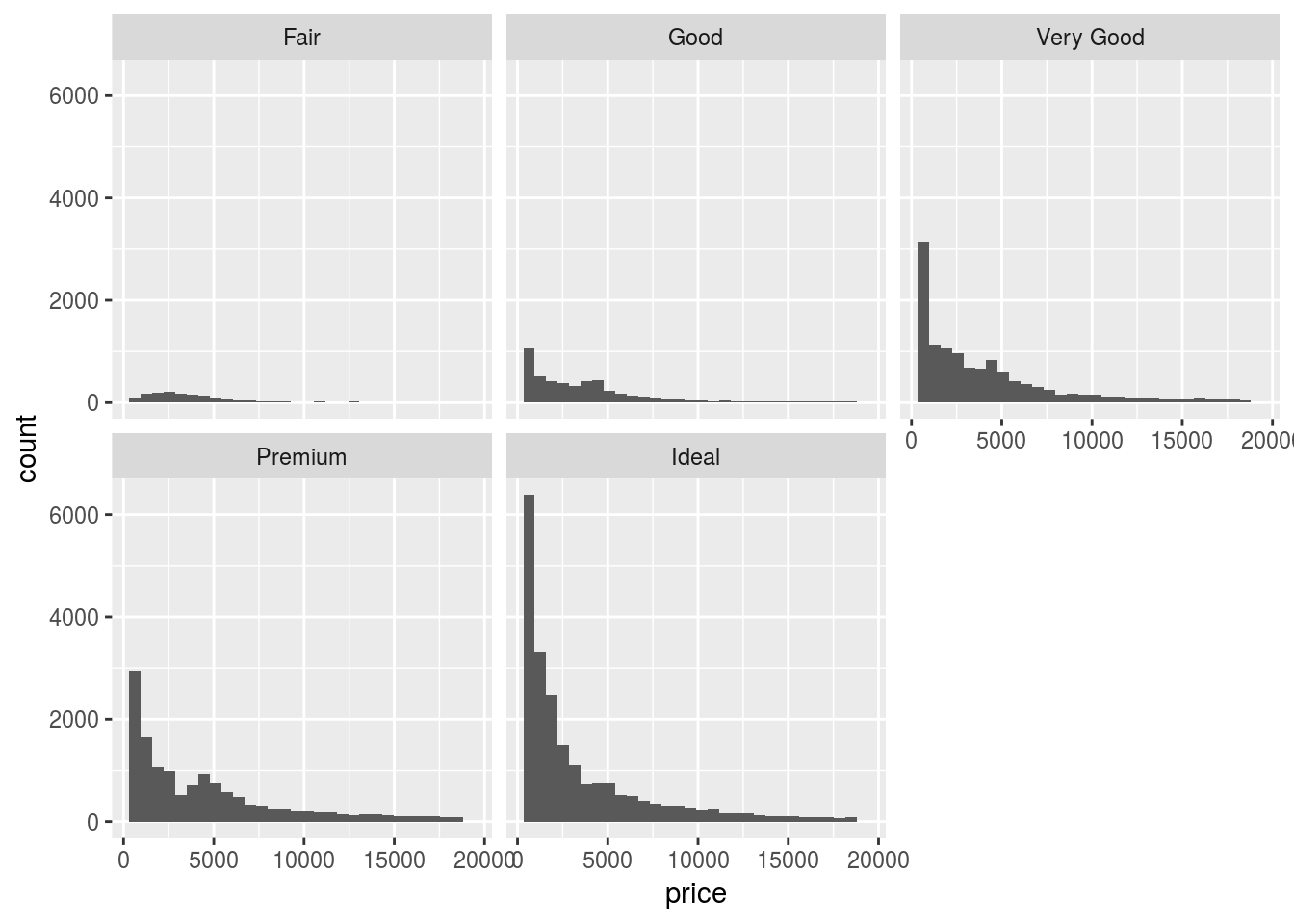``````ggplot(diamonds) +
geom_freqpoly(aes(x = price)) +
facet_wrap(~cut)``````
``## `stat_bin()` using `bins = 30`. Pick better value with `binwidth`.``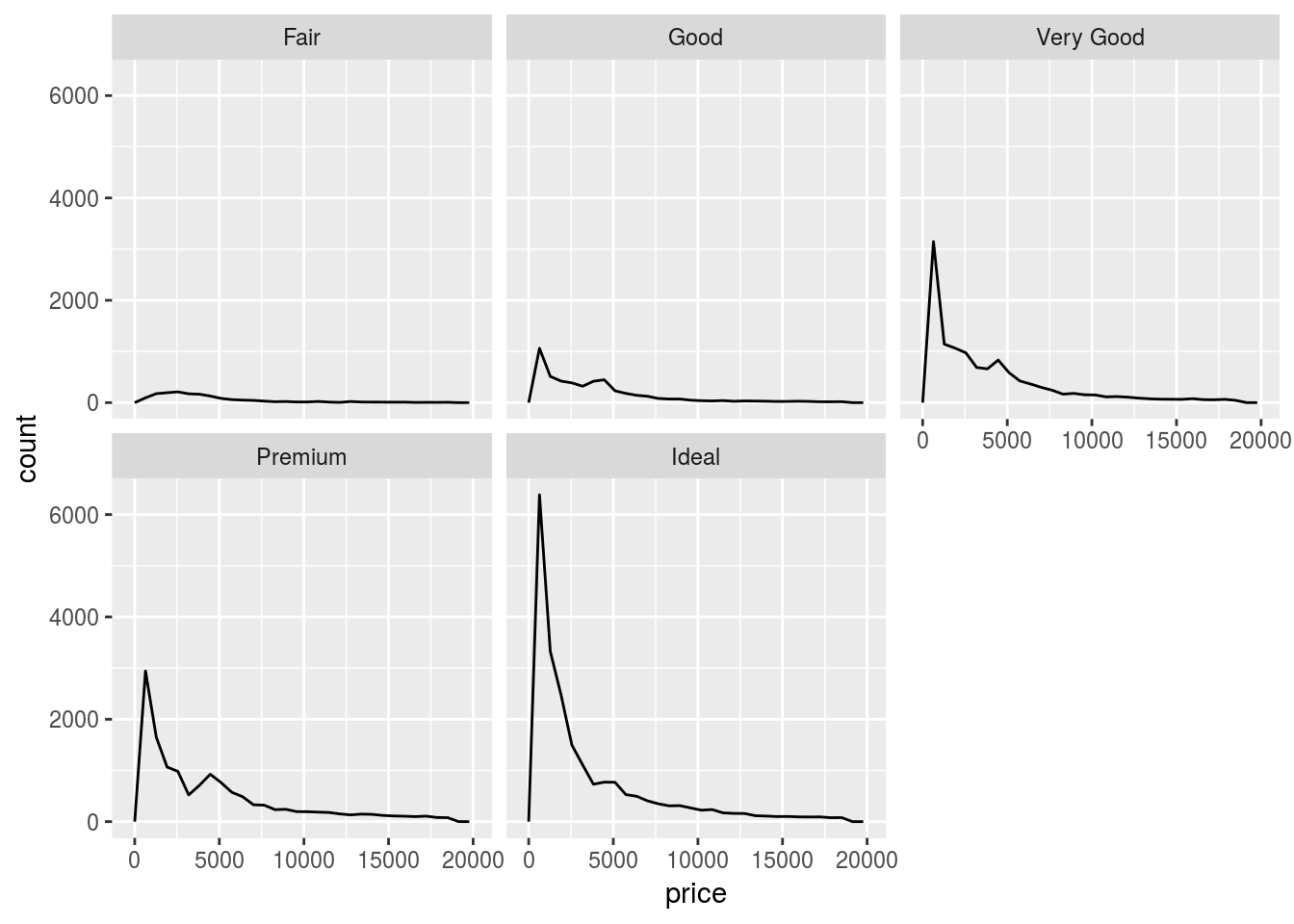``````ggplot(diamonds) +
geom_violin(aes(x = cut, y = price))``````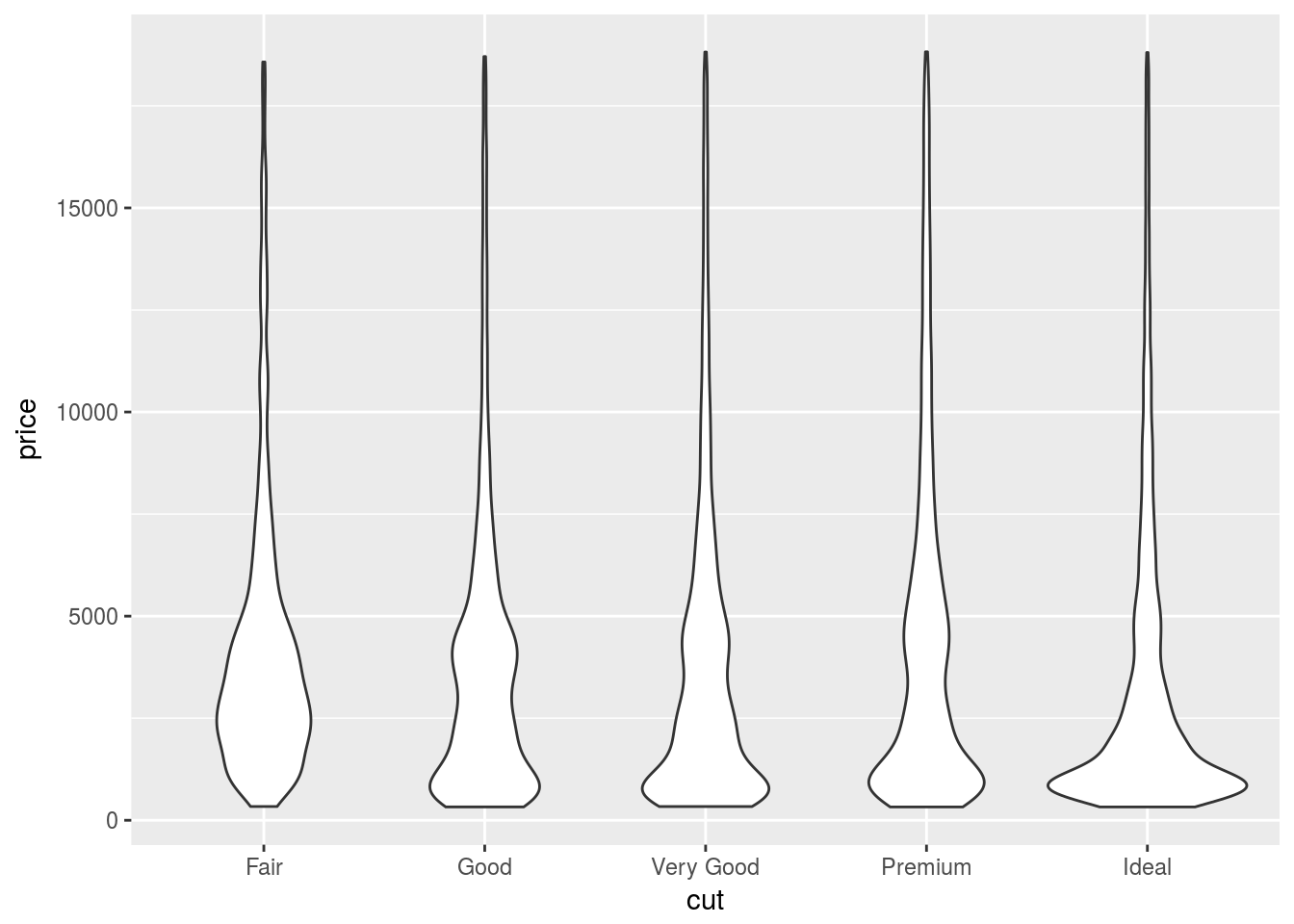``````ggplot(diamonds) +
geom_lv(aes(x = cut, y = price))``````Violin plot is best to compare the density distribution across different categories.

1. If you have a small dataset, it’s sometimes useful to use `geom_jitter()` to see the relationship between a continuous and categorical variable. The ggbeeswarm package provides a number of methods similar to `geom_jitter()`. List them and briefly describe what each one does.